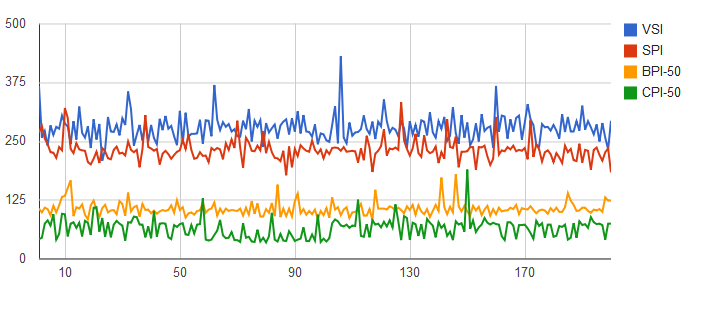## 2011/08/02

### Fast Inserts to PostgreSQL with JDBC and COPY FROM

I was reading some materials on how to make database inserts as efficient as possible from Java. it was motivated by an already existing application for storing of some measurements into PosgtreSQL. So I decided to compare known approaches to see if there is some way how to improve the application already using batched inserts.

For the purpose of  the test I created following table:

`CREATE TABLE measurement`
```(
measurement_id bigint NOT NULL,
valid_ts timestamp with time zone NOT NULL,
measurement_value numeric(19,4) NOT NULL,
CONSTRAINT pk_mv_raw PRIMARY KEY (measurement_id, valid_ts)
)
WITH (OIDS=FALSE)
```

I decided to test the insertion of 1000 records to the table. The data for the recors was generated before running of any of test methods. Four test methods were created to reflect ususal approaches:
• VSI (Very Stupid Inserts) - executing queries made of concatenated Strings one by one
• SPI  (Stupid Prepared Inserts) - similar to VSI but using prepared statements
• BPI (Batched Prepared Inserts) - prepared inserts, executed in batches of various length
• CPI (Copy Inserts) - inserts based on COPY FROM, executed in batches of various length
Prior to each inserts the table is cleared, the same after all data are succesfully inserted. Commit is called only once in each test method, following all the insert calls.  The following code exerpts illustrate the above listed approaches:

VSI

```for (int i=0; i<testSize; i++)
{
insertSQL = "insert into measurement values ("
+ measurementIds[i] +",'"+ timestamps[i] +"',"+values[i]+")";
insert.execute(insertSQL);
}
```

SPI
`PreparedStatement insert = conn.prepareStatement("insert into measurement values (?,?,?)");`
```for (int i=0; i<testSize; i++)
{
insert.setLong(1,measurementIds[i]);
insert.setTimestamp(2, timestamps[i]);
insert.setBigDecimal(3, values[i]);
insert.execute();
}
```

BPI

```PreparedStatement insert = conn.prepareStatement("insert into measurement values (?,?,?)");
```
```for (int i=0; i<testSize; i++)
{
insert.setLong(1,measurementIds[i]);
insert.setTimestamp(2, timestamps[i]);
insert.setBigDecimal(3, values[i]);
if (i % batchSize == 0) { insert.executeBatch(); }
}
insert.executeBatch();
```

CPI

```StringBuilder sb = new StringBuilder();
CopyManager cpManager = ((PGConnection)conn).getCopyAPI();
for (int i=0; i<testSize; i++)
{
sb.append(measurementIds[i]).append(",'")
.append(timestamps[i]).append("',")
.append(values[i]).append("\n");
if (i % batchSize == 0)
{
cpManager.copyIn("COPY measurement FROM STDIN WITH CSV", reader );
sb.delete(0,sb.length());
}
}Average time in millisecondsAll the 200 runs individually